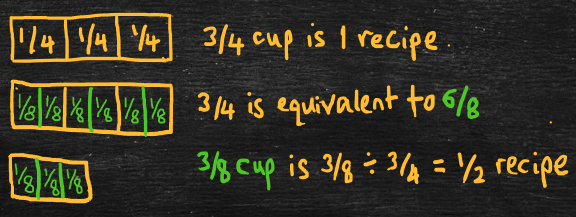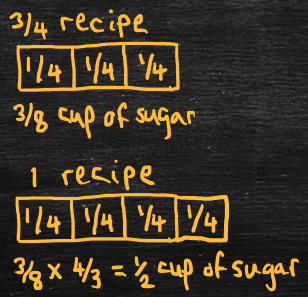Part 4: Division

# 4.5: Word problems for dividing fractions

Notes

In the following, “A/B” represents the fraction “A over B.” For example, “2/3” refers to the fraction “2 over 3” or “two-thirds.” Word problems for the division problem, A/B ÷ C/D, can either use the “how-many-groups” interpretation of division or the “how-many-units-in-one-group interpretation.

##### How many groups?

A/B ÷ C/D represents, “How many groups are there if A/B units are divided into groups of C/D units?” Another way to say this is, “How many times does C/D go into A/B?” For example,

• Arithmetic problem: Solve 3/8 ÷ 3/4.
• Word problem: A recipe calls for 3/4 of a cup of sugar, but Marybeth only has 3/8 of a cup of sugar. How much of the recipe can Marybeth make?
• Solve by expressing fractions using common denominators and dividing the numerators.
• 3/8 ÷ 3/4 = 3/8 ÷ 6/8 = 3 ÷ 6 = 1/2, so Marybeth can make 1/2 the recipe.
• Here, a recipe is a “group,” cups of sugar are the units, and we’re finding the fraction of a recipe we can make with 3/8 of a cup of sugar. In other words, 3/8 of a cup of sugar ÷ 3/4 of a cup of sugar per recipe = 1/2 a recipe.
• Equivalently, 1/2 a recipe times 3/4 of a cup of sugar per recipe equals 3/8 of a cup of sugar.Here’s another example,

• Arithmetic problem: Solve 2/3 ÷ 4/9.
• Word problem: Lina has read 2/3 of the textbook, while Mari has read 4/9 of the textbook. Proportionally, how much more of the textbook has Lina read than Mari?
• Solve by expressing fractions using common denominators and dividing the numerators.
• 2/3 ÷ 4/9 = 6/9 ÷ 4/9 = 6 ÷ 4 = 3/2, so Lina has read 3/2 or 1  1/2 (one and a half) times more of the textbook than Mari.

How many units in one group?

A/B ÷ C/D represents, “How many units are in one group if A/B units are divided into C/D groups?” To scale C/D groups to one group, multiply by D/C, so also scale A/B units by multiplying by D/C. For example,

• Arithmetic problem: Solve 3/8 ÷ 3/4.
• Word problem: Saima uses 3/8 of a cup of sugar to make 3/4 of a recipe. How much sugar is in a whole recipe?
• Solve using “invert and multiply.”
• 3/8 ÷ 3/4 = 3/8 x 4/3 = 1/2, so one recipe has 1/2 a cup of sugar.
• Again, a recipe is a “group” and the cups of sugar are the units, but now we’re finding the fraction of a cup of sugar in one recipe: 3/8 of a cup of sugar ÷ 3/4 of a recipe = 1/2 of a cup of sugar per recipe.
• Equivalently, 1/2 of a cup of sugar per recipe times 3/4 of a recipe equals 3/8 of a cup of sugar.Here’s another example:

• Arithmetic problem: Solve 2/3 ÷ 4/9.
• Word problem: Skylar fills 4/9 of a jar with 2/3 of a pound of jelly beans. How many pounds of jelly beans does Skylar need to fill the whole jar?
• Solve using “invert and multiply.”
• 2/3 ÷ 4/9 = 2/3 x 9/4 = 3/2, so Skylar needs 3/2 or 1  1/2 (one and a half) pounds of jelly beans to fill the whole jar.

The video below works through some examples of word problems for dividing fractions.

Practice Exercises

Do the following exercises to practice matching fraction division problems and word problems.